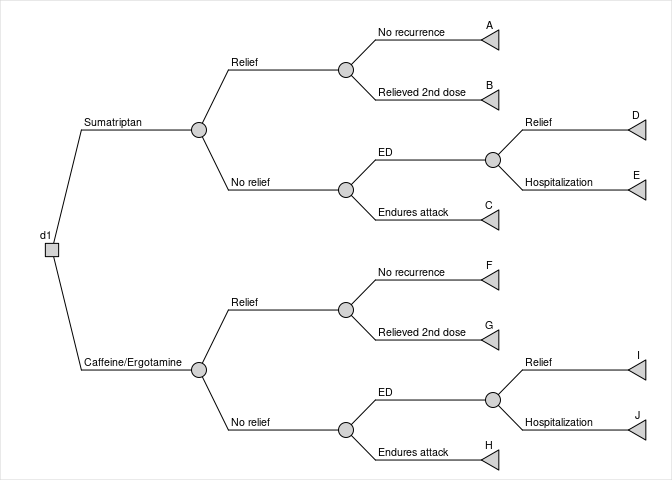# Introduction

This vignette is an example of modelling a decision tree using the rdecision package. It is based on the example given by Briggs1 (Box 2.3) which itself is based on a decision tree which compared oral Sumatriptan versus oral caffeine/Ergotamine for migraine2.

# Creating the model

## Constructing the tree

The following code constructs the decision tree. In the formulation used by rdecision, a decision tree is a form of arborescence, a directed graph of nodes and edges, with a single root and a unique path from the root to each leaf node. Decision trees comprise three types of node: decision, chance and leaf nodes and two types of edge: actions (whose sources are decision nodes) and reactions (whose sources are chance nodes), see figure.

# Time horizon
th <- as.difftime(48, units="hours")

# model variables for cost
c.sumatriptan <- 16.10
c.caffeine <- 1.32
c.ED <- 63.16

# Sumatriptan branch
ta <- LeafNode$new("A", utility=1.0, interval=th) tb <- LeafNode$new("B", utility=0.9, interval=th)
c3 <- ChanceNode$new() e1 <- Reaction$new(c3, ta, p=0.594, label="No recurrence")
e2 <- Reaction$new(c3, tb, p=0.406, cost=c.sumatriptan, label="Relieved 2nd dose") td <- LeafNode$new("D", utility=0.1, interval=th)
te <- LeafNode$new("E", utility=-0.3, interval=th) c7 <- ChanceNode$new()
e3 <- Reaction$new(c7, td, p=0.998, label="Relief") e4 <- Reaction$new(c7, te, p=0.002, cost=c.admission, label="Hospitalization")

tc <- LeafNode$new("C", utility=-0.3, interval=th) c4 <- ChanceNode$new()
e5 <- Reaction$new(c4, tc, p=0.920, label="Endures attack") e6 <- Reaction$new(c4, c7, p=0.080, cost=c.ED, label="ED")

c1 <- ChanceNode$new() e7 <- Reaction$new(c1, c3, p=0.558, label="Relief")
e8 <- Reaction$new(c1, c4, p=0.442, label="No relief") # Caffeine/Ergotamine branch tf <- LeafNode$new("F", utility=1.0, interval=th)
tg <- LeafNode$new("G", utility=0.9, interval=th) c5 <- ChanceNode$new()
e9 <- Reaction$new(c5, tf, p=0.703, label="No recurrence") e10 <- Reaction$new(c5, tg, p=0.297, cost=c.caffeine,
label="Relieved 2nd dose")

ti <- LeafNode$new("I", utility=0.1, interval=th) tj <- LeafNode$new("J", utility=-0.3, interval=th)
c8 <- ChanceNode$new() e11 <- Reaction$new(c8, ti, p=0.998, label="Relief")
e12 <- Reaction$new(c8, tj, p=0.002, cost=c.admission, label="Hospitalization") th <- LeafNode$new("H", utility=-0.3, interval=th)
c6 <- ChanceNode$new() e13 <- Reaction$new(c6, th, p=0.920, label="Endures attack")
e14 <- Reaction$new(c6, c8, p=0.080, cost=c.ED, label="ED") c2 <- ChanceNode$new()
e15 <- Reaction$new(c2, c5, p=0.379, label="Relief") e16 <- Reaction$new(c2, c6, p=0.621, label="No relief")

# decision node
d1 <- DecisionNode$new("d1") e17 <- Action$new(d1, c1, cost=c.sumatriptan, label="Sumatriptan")
e18 <- Action$new(d1, c2, cost=c.caffeine, label="Caffeine/Ergotamine") # create lists of nodes and edges V <- list( d1, c1, c2, c3, c4, c5, c6, c7, c8, ta, tb, tc, td, te, tf, tg, th, ti, tj ) E <- list( e1, e2, e3, e4, e5, e6, e7, e8, e9, e10, e11, e12, e13, e14, e15, e16, e17, e18 ) # tree DT <- DecisionTree$new(V,E)

## Tree diagramDecision tree for the Sumatriptan model

# Running the model

The method evaluate of decision tree objects computes the probability, cost and utility of each strategy for the model. A strategy is a unanimous prescription of the actions at each decision node. In this example there is a single decision node with two actions, and the strategies are simply the two forms of treatment to be compared. More complex decision trees are also possible.

The paths traversable in each strategy can be evaluated individually using the method evaluate(by="path"). In rdecision a strategy is defined as a set of action edges with one action edge per decision node. It is necessary to use the option by="path" only if information about each pathway is required; normally it is sufficient to call evaluate which will automatically aggregate the evaluation by strategy.

# Model results

The evaluation of each pathway, for each strategy, yields the following table:

Leaf d1 Probability Cost Utility QALY
A Sumatriptan 0.33 5.34 0.33 0.0018
B Sumatriptan 0.23 7.29 0.20 0.0011
C Sumatriptan 0.41 6.55 -0.12 -0.0007
D Sumatriptan 0.04 2.80 0.00 0.0000
E Sumatriptan 0.00 0.08 0.00 0.0000
F Caffeine/Ergotamine 0.27 0.35 0.27 0.0015
G Caffeine/Ergotamine 0.11 0.30 0.10 0.0006
H Caffeine/Ergotamine 0.57 0.75 -0.17 -0.0009
I Caffeine/Ergotamine 0.05 3.20 0.00 0.0000
J Caffeine/Ergotamine 0.00 0.12 0.00 0.0000

There are, as expected, ten pathways (5 per strategy). The expected cost, utility and QALY (utility multiplied by the time horizon of the model) for each choice can be calculated from the table above, or by invoking the evaluate method of a decision tree object with the default parameter by="strategy". This gives the following result, consistent with that reported by Evans et al2.

d1 Cost Utility QALY
Caffeine/Ergotamine 4.71 0.20128 0.00110
Sumatriptan 22.06 0.41686 0.00228